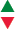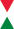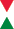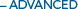# System Throughput, f/#, and Numerical Aperture

Authors: Gregory Hollows, Nicholas James

This is Section 2.1 of the Imaging Resource Guide.

The f/# (pronounced “F-number”) setting on a lens controls overall light throughput, depth of field (DOF), and the ability to produce contrast at a given resolution. Fundamentally, f/# is the ratio of the focal length, $\small{\left( f \right)}$,  of the lens to the effective aperture diameter $\small{ \left( \varnothing _{\text{EA}} \right) }$:

(1)$$\text{f}/\# = \frac{f}{ \varnothing _{\text{EA}}}$$
(1)
$$\text{f}/\# = \frac{f}{Ø_{\text{EA}}}$$

In most lenses, the f/# is set by turning the iris adjusting ring (See The Anatomy of a Lens). This movement opens and closes the iris diaphragm inside. The numbers labeling the ring denote light throughput associated with the aperture diameter and usually increase by multiples of $\sqrt{2}$. Increasing the f/# by a factor of $\sqrt{2}$ will halve the aperture area, decreasing the light throughput of the lens by a factor of 2. Lenses with lower f/#s are considered fast and allow more light to pass through the system, while lenses of higher f/#s are considered slow and feature reduced light throughput. Table 1 shows an example of f/#, aperture diameters, and effective opening sizes for a 25mm focal length lens. Notice that from the setting of f/1 to f/2, and again for f/4 to f/8, the lens aperture is reduced by half and the effective area is reduced by a factor of 4 at each interval. This illustrates the reduction in throughput associated with increasing a lens’s f/#.

f/#Lens Aperture Diameter (mm)Aperture Opening Area (mm2)
1 25.0 490.8
1.4 17.9 251.6
2 12.5 122.7
2.8 8.9 62.2
4 6.3 31.2
5.6 4.5 15.9
8 3.1 7.5

Table 1: The relationship between f/# and effective area for a 25mm singlet lens. As the f/# increases, the area decreases, leading to a slower system with less light throughput.

### f/# and Effects on a Lens’s Theoretical Resolution, Contrast, DOF

The f/# impacts more than just light throughput. Specifically, f/# is directly related to the theoretical resolution, contrast limits, and the depth of field (DOF) and depth of focus of the lens (See Depth of Field and Depth of Focus for more information about DOF). The f/# also influences the aberrations of a specific lens design. As pixel size decreases, f/# becomes one of the most important factors of system performance because f/# drives DOF and resolution in opposite directions. As shown in Table 2, the requirements are often in direct conflict and compromises must be made. These tradeoffs are discussed in more detail later on in this section.

f/#Diffraction Limited ResolutionDepth of FieldLight ThroughputNumerical ApertureTable 2: Lens performance changes as the f/# varies.

## f# Changes with Working Distance Change### f/# Changes with a Working Distance Change

The definition of f/# in Equation 1 is limited; f/# is defined at an infinite working distance (WD) where magnification is effectively zero. In most machine vision applications, the object is located much closer to the lens than infinitely far away. As such, working F-number, (f/#)w, seen in Equation 2, is a more useful representation of f/# in most applications.

(2)$$\left( \text{f}/ \# \right)_w \approx \left( 1 + m \right) \times \left( \text{f}/ \# \right)$$
(2)
$$\left( \text{f}/ \# \right)_w \approx \left( 1 + m \right) \times \left( \text{f}/ \# \right)$$

In the equation for(f/#)w, m represents the magnification (ratio of image to object height) of the lens. Note that as m approaches zero (as the object approaches infinity), (f/#)w approaches f/#. It is especially important to keep (f/#)w in mind at smaller WDs. For example, an f/2.8, 25mm focal length lens operating with a magnification of 0.5X will have an effective (f/#)w of f/4.2. This impacts image quality as well as the lens’s ability to collect light.

### f/# and Numerical Aperture

It can often be easier to talk about the overall light throughput as the cone angle, or the numerical aperture (NA), of a lens. The NA of a lens is defined as the sine of the angle made by the marginal ray and optical axis in image space, shown in Figure 1.##### Figure 1: Visual representation of f/#, both for a simple lens (a), and a real-world system (b).

It is important to remember that f/# and NA are inversely related.

(3)$$\text{NA} = \frac{1}{2 \times \left( \text{f}/\# \right)}$$
(3)
$$\text{NA} = \frac{1}{2 \times \left( \text{f}/\# \right)}$$

Table 3 shows a typical f/# layout on a lens (each f/# value increasing by a factor of $\sqrt{2}$ along with its NA.

 f/# 1.4 2 2.8 4 5.6 8 11 16 NA 0.36 0.25 0.18 0.13 0.09 0.06 0.05 0.03

Table 3: Relationship between f/# and numerical aperture.

Light throughput is typically referred to as NA instead of f/# in microscopy, but it is important to note that the NA values that are specified for microscope objectives are specified in object space. More information about how f/# affects resolution can be found in the sections on the modulation transfer function (MTF), the diffraction limit, and the Airy disk. Details on f/# and DOF can be found Depth of Field and Depth of Focus.

Featured Resources